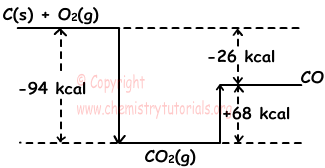## Hess's Law (Summation of Thermochemical Reactions)

Hess' Law (Summation of Thermochemical Reactions)

Hess' law states that, you can sum one more than one reactions to form new reaction. While doing this, you apply same changes also on enthalpy changes of used reactions. Following examples shows Hess' law in detail.

Example: Use reactions given below;

I. C(s) + O2(g) → CO2(g) ; ∆H=-94 kcal

II. 2CO(g) + O2(g) → 2CO2(g) ; ∆H=-136 kcal

to get enthalpy change of following reaction.

C(k) + 1/2O2(g) → CO(g) ; ∆H=?

Solution:

In chemical reactions, you can sum each sides of reactions like mathematical equations. We sum reactions I and II, but result does not give us what question asks. Thus, we should do some other calculations to get wanted reaction. If we reverse reaction II and multiply it 1/2 before adding reaction I, we get wanted reaction.

I. C(s) + O2(g) → CO2(g) ; ∆H=-94 kcal

II. CO2(g)  → CO(g) + 1/2O2(g) ; ∆H=-1/2(-136) kcal  ("-" in front of ∆H result of reversing it and we multiply reaction with 1/2)

¯¯¯¯¯¯¯¯¯¯¯¯¯¯¯¯¯¯¯¯¯¯¯¯¯¯¯¯¯¯¯¯¯¯¯¯¯¯¯¯¯¯

C(k) + 1/2O2(g) → CO(g) ; ∆H=-94+68=-26 kcal

We get reaction above by using Hess' law. Schema given below summarizes this process;Example: Using given thermochemical reactions;

I. NO2(g) + 13,5 kcal → NO(g) + 1/2O2(g)

II. 2NO(g) → N2(g) + O2(g) + 43 kcal

III. N2(g) + 2O2(g) + 2 kcal → N2O4(g)

find enthalpy change of following reaction;

N2O4(g) → 2NO2(g)

Solution:

Reaction III is reversed ( or multiply -1)

N2O4(g) → N2(g) + 2O2(g) + 2 kcal

Reaction I is reversed and multiplied by 2 (or multiplied by -2)

2NO(g) + O2(g) → 2NO2(g) + 2.(13,5) kcal

Final reaction does not consist of NO given in first reaction. Thus, we reverse reaction II (or multiply by -1)

N2(g) + O2(g) + 43 kcal → 2NO(g)

We sum these three reactions;

N2O4(g) → N2(g) + 2O2(g) + 2 kcal

2NO(g) + O2(g) → 2NO2(g) + 27 kcal

N2(g) + O2(g) + 43 kcal → 2NO(g)

¯¯¯¯¯¯¯¯¯¯¯¯¯¯¯¯¯¯¯¯¯¯¯¯¯¯¯¯¯¯¯

N2O4(g) + 14 kcal → 2NO2(g)

Enthalpy change of wanted reaction is 14 kcal.

Enthalpy concept is used for all chemical reactions. But, there are some special reactions like formation combustion. We can give them specific name and their enthalpies are also have specific names. Some of them are given below;

1) Standard Molar Enthalpy of Formation:

Enthalpy change of formation of 1 mole compound from its elements is called standard molar enthalpy of formation and expressed in kcal/mol or kjoule/mol. Since enthalpy of reactions change with temperature and pressure, pressure and temperature must be constant. Standard values of temperature is 25 0C and pressure is 1 atm. Standard formation enthalpy of element for its stable conditions is assumed to be zero. For example, C has two allotrope graphite and diamond, under standard conditions, graphite is more stable than diamond, so standard formation enthalpy of graphite is zero but standard enthalpy of diamond is different from zero. Be careful when writing formation reactions an pay attention on following suggestions;

• Reaction must be written for 1 mole compound
• Compound must be formed by elements
• Compound must be formed stable elements

Examples:

1) C(graphite) + O2(g) → CO2(g) ; ∆H=-94 kcal/mol

This reaction is formation reaction. Enthalpy of formation of CO2 is -94 kcal and we express it,

∆HF(CO2.g)=-94 kcal

2) C(diamond) + O2(g) → CO2(g) ; ∆H=-94,5 kcal

This reaction is not formation reaction since C(diamond) is not stable form of C.

3) H2(g) + I2(g) → 2HI(g) ; ∆H=12,4 kcal

This reaction is not formation reaction since 2 mol compound are formed.

If we know formation enthalpy of matters, we can find ∆H value of reactions.

Reactants → Products ; ∆H=?

We find ∆H by following equation;

∆H=Σa∆H(F.(Products) - Σb∆H(F.(Reactants)

Where a and b are coefficients of matters in reaction. For example;

aA + bB → cC + dD

Enthalpy of this reaction is found by ;

∆H=[c∆H(F.(C) + d∆H(F.(D)] - [a∆H(F.(A) + b∆H(F.(B)]

2) Standard Enthalpy of Decomposition:

Enthalpy change of decomposition of  1 mole compound into its elements is called standard molar enthalpy of decomposition.

Example:

H2O(l) → H2(g) + 1/2 O2(g) ; ∆H=68 kcal

Standard molar enthalpy of H2O (l) is 68 kcal.

3) Standard Enthalpy of Combustion:

It is the heat released from the reaction of one mole element with O2(g).

Example:

CH4(g) + 2O2(g) → CO2(g) + 2H2O(l) ; ∆H=-212 kcal

Molar enthalpy of combustion of CH4(g) is -212 kcal. Most of the combustion reactions are exothermic.

Example: Heat released from the reaction of formation P2O5 from elements P and O2 depend on which one of the following quantities;

I. Using white or red phosphor

II. Using Oxygen or ozone gas

III. Number of mole of P2O5

Solution:

I. White and red phosphor are allotrope of phosphor element. Thus, they have different enthalpy. It changes  ∆H of P2O5.

II. Oxygen and ozone are allotrope of oxygen element. Thus, they have different enthalpy. It changes  ∆H of P2O5.

III. Enthalpy of P2O5 increases with increasing mole.

4) Standard Enthalpy of Neutralization Reaction:

It is the enthalpy change of neutralization of 1 mol acid and one mol base. These reactions are exothermic reactions.

Acid + Base → Salt + Water + Heat

Example:

H+ + OH- → H2O + 13,5 kcal

Molar neutralization enthalpy is -13,5 kcal

Author:

Tags:

© Copyright www.ChemistryTutorials.org, Reproduction in electronic and written form is expressly forbidden without written permission of www.ChemistryTutorials.org. Privacy Policy